Download Presentation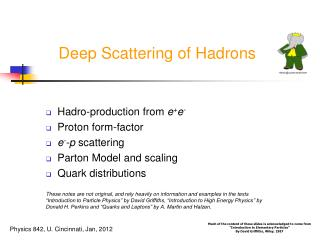Deep Scattering of Hadrons

# Deep Scattering of Hadrons - PowerPoint PPT Presentation

Deep Scattering of Hadrons. Hadro-production from e + e - Proton form-factor e - -p scattering Parton Model and scaling Quark distributionsI am the owner, or an agent authorized to act on behalf of the owner, of the copyrighted work described.
Download Presentation## Deep Scattering of Hadrons

An Image/Link below is provided (as is) to download presentation

Download Policy: Content on the Website is provided to you AS IS for your information and personal use and may not be sold / licensed / shared on other websites without getting consent from its author.While downloading, if for some reason you are not able to download a presentation, the publisher may have deleted the file from their server.

- - - - - - - - - - - - - - - - - - - - - - - - - - E N D - - - - - - - - - - - - - - - - - - - - - - - - - -
Presentation Transcript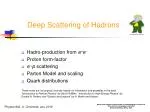### Deep Scattering of Hadrons

Proton form-factor

e--p scattering

Parton Model and scaling

Quark distributions

These notes are not original, and rely heavily on information and examples in the texts “Introduction to Particle Physics” by David Griffiths, “Introduction to High Energy Physics” by Donald H. Perkins and “Quarks and Leptons” by A. Martin and Halzen.

Much of the content of these slides is acknowledged to come from

“Introduction to Elementary Particles”

By David Griffiths, Wiley, 1987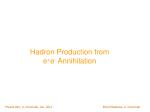e+e- Annihilation

Brian Meadows, U. Cincinnati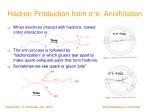_

e+e-qq

Time

Time

_

e+e-qqg

Hadron Production from e+e- Annihilation
• When electrons interact with hadrons, lowest order interaction is:
• The e/m process is followed by “hadronization” in which gluons tear apart to make quark-antiquark pairs that form hadrons.
• Sometimes we see quark or gluon “jets”

Brian Meadows, U. Cincinnati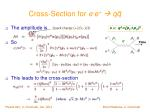Cross-Section for e-e+ qq
• The amplitude is
• So
• This leads to the cross-section

s ´ q2=(p1+p2)2

Quark charge (+2/3,-1/3)

p1

p3

q

Time

p2

p4

Brian Meadows, U. Cincinnati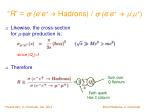“R” = s (e-e+ Hadrons) / s (e-e+m-m+)
• Likewise, the cross-section for m-pair production is:

since |Qm|=1

• Therefore

Sum over

Q flavours

Each quark

Has 3 colours

Brian Meadows, U. Cincinnati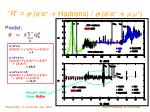“R” = s (e-e+ Hadrons) / s (e-e+m-m+)

Predict:

u+d+s:

3 [(2/3) 2 + (-1/3) 2 + (-1/3) 2]

= 2

u+d+s+c:

3 [(2/3) 2 + (-1/3) 2 + (-1/3) 2

+ (2/3) 2]

= 10/3

u+d+s+c+b:

3 [(2/3) 2 + (-1/3) 2 + (-1/3) 2

+ (2/3) 2 + (-1/3) 2]

= 11/3

Recent data

fromBaBar

Brian Meadows, U. Cincinnati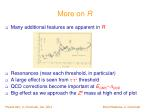More on R
• Many additional features are apparent in R
• Resonances (near each threshold, in particular)
• A large effect is seen from t-t+ threshold
• QCD corrections become important at ECMS~LQCD
• Big effect as we approach the Z0 mass at high end of plot

Brian Meadows, U. Cincinnati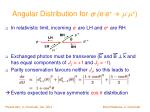Angular Distribution for s (e-e+m-m+)
• In relativistic limit, incoming e- are LH and e+ are RH
• Exchanged photon must be transverse (E and B ? k and has equal components of Jz = +1 and Jz = -1).
• Parity conservation favours neither Jz, so this leads to

 Events expected to have symmetric cos  distribution

+

RH

LH

e +

e -

LH

RH

 -

Brian Meadows, U. Cincinnati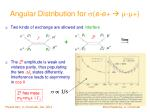Time

Angular Distribution for s(e-e+ -+)
• The Z0 amplitude is weak and violates parity, thus populating the two DJz states differently. Interference leads to asymmetry in cos 
• Two kinds of exchange are allowed and interfere

p1

p3

-

p1

p3

-

Z0

+

+

+

p2

p4

p2

p4

s/ 1/s

Z0 has mass

mZ=m0+ i GZ

Brian Meadows, U. Cincinnati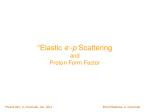“Elastic e--p Scattering

and

Proton Form Factor

Brian Meadows, U. Cincinnatiq

q

Time

Elastic e--p Scattering
• e--p scattering is like e-m scattering if p is point-like
• For e-m scattering we obtained:

e -

e -

e -

e -

p form-factor

m

m

p

p

e- mass

Brian Meadows, U. Cincinnatiq

q

Time

Proton Form-Factor
• e - ’s (or  ’s) can be used to “probe” inside the proton
• As a (virtual)  does the probing, we anticipate both electric and a magnetic form-factors, GE(q2) and GM(q2)
• Factorizing the two vertices:

e -

e -

e -

e -

p form-factor

m

m

p

p

Brian Meadows, U. CincinnatiProton Form-Factor
• K must be a symmetric, rank 2 tensor, as is L
• It is formed from p = p2 and q = p4 - p2 = p3 – p1
• It can be shown (homework) that this must be written as

Anti-symmetric

Brian Meadows, U. CincinnatiProton Form-Factor
• First we note that qmL= 0 :
• Using q = p1 – p3 :

(since p12 = p32 = m2c2).

• This implies, that qmK= 0 also … (though not obvious).

Brian Meadows, U. CincinnatiProton Form-Factor
• Evaluating qmK:
• If this is zero for any p or q, then

Using p¢q = -q2 / 2 we obtain:

Brian Meadows, U. CincinnatiProton Form-Factor
• Therefore we write K as:
• Now evaluate
• So that

Brian Meadows, U. CincinnatiProton Form-Factor
• Evaluate the cross-section in the lab frame where

and we neglect m (<< M)

to obtain (more homework!) – the “Rosenbluth formula”:

• Simplest model (point-like proton)  Mott scattering:

K1 = -q2 ; K2 = 4M2c2

GM(q 2)

GE(q 2)

Brian Meadows, U. CincinnatiProton Form-Factor
• Next simplest form that works to larger q2 is

“MV”~ 0.9 GeV/c2

Brian Meadows, U. CincinnatiProton Form-Factor
• Best data so far – from e+e- p p atBaBar

Brian Meadows, U. CincinnatiProton Form-Factor
• BaBar was, for first time, able to distinguish GE from GM

Brian Meadows, U. Cincinnatiq

“Inclusive” (Inelastic) e--p Scattering
• For elastic scattering, q2 depends on E and :

where we neglect m (<< M)

• A better probe is to use all inelastic channels:
• Typically, record only p1 and p3 (e- in and e- out)

electron lab. energies E1 =E and E3 = E0 respectively.

p3

p1

E’

e -

e -

E

p form-factor

p2 =p

p

X (p4, …pn)

Brian Meadows, U. Cincinnatiq

q

Time

Elastic Scattering

Inelastic Scattering

• E’determined by E and
• Invariant mass MX = Mp
• Only one invariant q

q2 = 2MX(E`-E)

p¢ q = -q2/2

• E’notdetermined by E and alone, but

E’´ E’ (E, , MX)

• MXis a free variable = Mp
• Two invariants: q

q2 = 2MX(E`-E) - Mp2 + Mx2

Bjorken x (=1 for elastic case)

E’

e -

e -

E’

E

E

e -

e -

E, p

E’, p’

p

X (p4, …pn)

Mp

Mp

Mp

p

p

MX

Brian Meadows, U. Cincinnatiq

Time

“Infinite Momentum System” (IMS)
• Proton momentum is very large:
• Neglect M (proton), m (electron) and mi (“parton”)
• Neglect transverse momentum components of partons
• All partons of type i carry momentum xi P
• Important kinematic quantities:
• Collision of parton with photon:

p3

p1

E

E 0

P

P

W = MX

Brian Meadows, U. Cincinnati“Inclusive” (Inelastic) e--p Scattering
• We can compute the cross-section, as before, but more complicated as we integrate over all X phase space:

for mass-less e -,in proton rest frame, where:

• Integration leads to

All possible

X ’s

Not determined

by E and 

Brian Meadows, U. Cincinnati“Inclusive” (Inelastic) e--p Scattering
• Proceed as before (replace K’s by W’s):
• As X has continuous mass distribution, the W ’s depend on two (not one) invariants:

Chooseq2andx = - 0.5 q2/ p¢ q

• Compare with elastic scattering (where x = 1):

To get Rosenbluth formula, we need:

In-elastic structure functions

W1,2´W 1,2(q2, x)

“Bjorken x”

Brian Meadows, U. CincinnatiBjorken Scaling
• Bjorken predicted that q2 dependence of W’s would vanish at high energy (IMS).

Define:

• This prediction was based on the idea that, as q2 increases, the virtual photon penetrates deeper into the proton to reveal individual “partons” (i.e. quarks).
• At this level, then, the scattering becomes g-q scattering and the q2-dependence vanishes.

Brian Meadows, U. CincinnatiBjorken Scaling
• Callen and Gross computed what is expected for g-q scattering and predicted that F1(x) and F2(x) were related
• for spin ½ partons

[ “ “ 0 “ ]

• Both these relationships are seen to be obeyed in the data
• Are predicted if “partons” of spin ½ exist
• Feynman (1969) concludes that partons ARE quarks

Brian Meadows, U. CincinnatiBjorken Scaling & Callen-Gross Relation

nW2 (¼ F2) vs. q2

at x = 0.25

(Friedman & Kendall, 1972)

For perfect “scaling” there is no

q2-dependence.

2xW1/F2vs. q2 /(2Mn)

For spin ½ partons, this is equal to 1.0.

(For spin 0 it is = 0)

1.0

1.5

1.0

0.5

0

2xF1/F2

0.5

0.4

0.3

0.2

0.1

0

n W2

0 2 4 6 8

0 0.5 1.0

q2 (GeV/c2) 2

x = q2/(2Mn)

Brian Meadows, U. CincinnatiQuark Distribution Functions
• The “parton model” views the proton (and all hadrons) as made up from Dirac (point-like) quarks and by gluons.
• Each quark or gluon has a distribution of momentum

p = x P (P is momentum of parent hadron)

inside the hadron given by a distribution function f(x) with

• The probability that the momentum of the quark (or gluon) lies between x and x+dx is f(x)dx .

Brian Meadows, U. CincinnatiThe Parton Model
• The physical reason for the Bjorken relations arise from the picture that exchanged photons scatter from individual, point-like quarks with charges Qi so that
• If a proton is made only of 2u and 1d“valence quarks” then:

with

Analogous to

Rosenbluth scattering

Brian Meadows, U. CincinnatiThe Parton Model
• In practice, quarks interact through gluons, and q-q pairs are sometimes made so we also expect a “sea” of all other quark and anti-quark types.
• Anticipating that all “sea” quarks have same distributions
• So

with terms for c, b and t quarks that are small due to their large masses (small propagators).

Brian Meadows, U. CincinnatiN 3 GeV

1.0

0.1

F2(x)

e-d 15 GeV

N 100 GeV

0 0.25 0.50 0.75 1.00

x

Structure Function F2(x)

Structure function F2(x) for various beam energies

Of e-N and n N scattering experiments. (N is

a nucleon)

The shrinkage is apparent as the beam energy

increases.

x 3 GeV nN - Gargamelle

(bubble chamber at CERN)

15 GeV e- d - SLAC

(fixed target experiment)

100 GeV nN - CDHS at Fermilab

(fixed target experiment)

Brian Meadows, U. Cincinnati